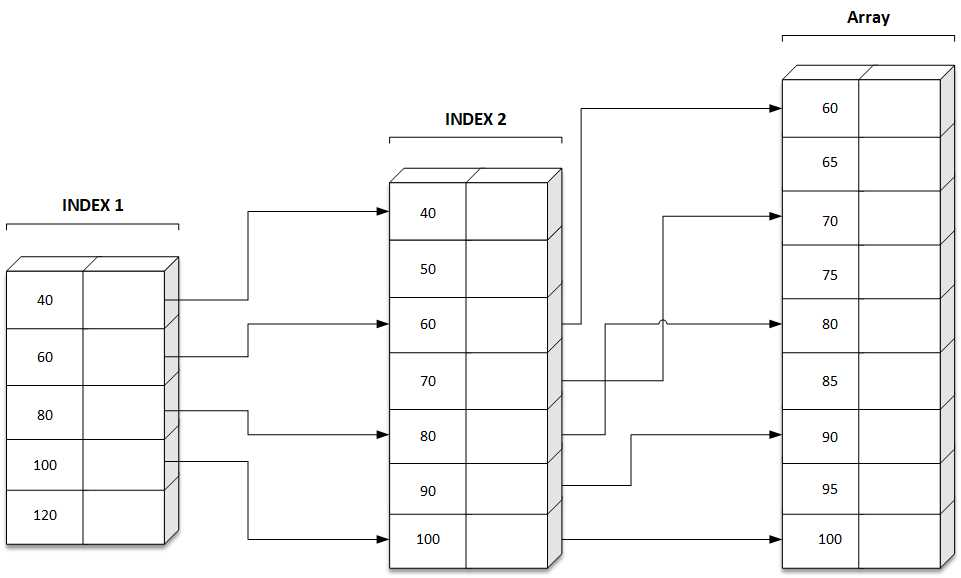# Array C Programing

Answer: Index search is special search. This search method is used to search a record in a file. Searching a record refers to the searching of location loc in memory where the file is stored. Indexed search searches the record with a given key value relative to a primary key field. This search method is accomplished by the use of pointers.

Index helps to locate a particular record with less time. Indexed sequential files use the principal of index creation.

For Example, Directory, book etc. In this type of file organization, the records are organized in a sequence and an index table is used to speed up the access to records without searching the entire file. The records of the file may be sorted in random sequence but the index table is in started sequence on the key field. Thus the file can be processed randomly as well as sequentially.

• More efficient.
• Time required is less.

"Sequential indexed search" diagram explanation:Code:

``````
// C program for Indexed Sequential Search
#include
#include

void indexedSequentialSearch(int arr[], int n, int k)
{
int elements, indices, temp, i;
int j = 0, ind = 0, start, end;
for (i = 0; i < n; i += 3) {

// Storing element
elements[ind] = arr[i];

// Storing the index
indices[ind] = i;
ind++;
}
if (k < elements) {
exit(0);
}
else {
for (i = 1; i <= ind; i++)
if (k < elements[i]) {
start = indices[i - 1];
end = indices[i];
break;
}
}
for (i = start; i <= end; i++) {
if (k == arr[i]) {
j = 1;
break;
}
}
if (j == 1)
printf("Found at index %d", i);
else
}

// Driver code
void main()
{

int arr[] = { 6, 7, 8, 9, 10 };
int n = sizeof(arr) / sizeof(arr);

// Element to search
int k = 8;
indexedSequentialSearch(arr, n, k);
}

``````

Output:

``````
Found at index 2
``````